The Rocscience International Conference 2021 Proceedings are now available. Read Now

# Shear Strength

In UnWedge, three different strength models are available for defining the shear strength of the joints used in the analysis:

• Mohr-Coulomb
• Barton-Bandis
• Power Curve

NOTE: For any of these strength models, a waviness angle can also be defined, which will have the effect of increasing the shear strength. See the Waviness Angle topic for details. If water pressure is included, then all parameters should be considered effective stress parameters. See the Water Pressure topic for details about including water pressure in the analysis.

## Mohr-Coulomb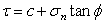Eqn.1

Whereis the friction angle of the joint plane and c is the cohesion.

### TENSILE STRENGTH

For the Mohr-Coulomb model, you may also define a tensile strength. If defined, the tensile strength will be applied to wedge faces which are in tension (for example: all planes of a falling wedge, or the non-sliding plane(s) of a sliding wedge). The tensile strength will apply a passive resisting force normal to the wedge plane, equal to the area of the wedge plane multiplied by the tensile strength.

## Barton-Bandis

The original Barton equation for the shear strength of a rock joint is given by Eqn.2a: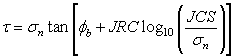Eqn.2a

Whereis the basic friction angle of the failure surface, JRC is the joint roughness coefficient, and JCS is the joint wall compressive strength [Barton, 1973, 1976]. On the basis of direct shear test results for 130 samples of variably weathered rock joints, Barton and Choubey revised this to Eqn.2b: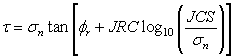Eqn.2b

Where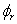is the residual friction angle of the failure surface [Barton and Choubey, 1977]. Barton and Choubey suggest thatcan be estimated from Eqn.2c:Eqn.2c

Where r is the Schmidt hammer rebound number on wet and weathered fracture surfaces and R is the Schmidt rebound number on dry unweathered sawn surfaces. Equations 2b and 2c have become part of the Barton-Bandis criterion for rock joint strength and deformability [Barton and Bandis, 1990].

For further information on the shear strength of discontinuities, including a discussion of the Barton-Bandis failure criterion parameters, see Practical Rock Engineering: Shear Strength of Discontinuities) on the Rocscience website.

## Power Curve

The Power Curve model for shear-strength [Miller, 1988], can be expressed as: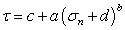Eqn.3

Where a, b and c are parameters typically obtained from a least-squares regression fit of data obtained from small-scale shear tests. The d parameter represents the tensile strength. If included, it must be entered as a positive value.